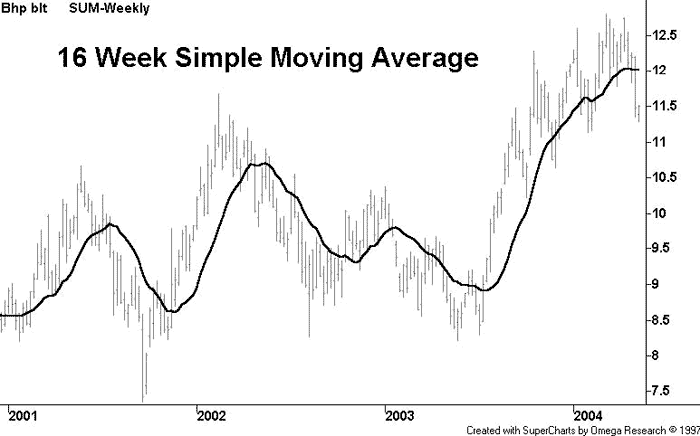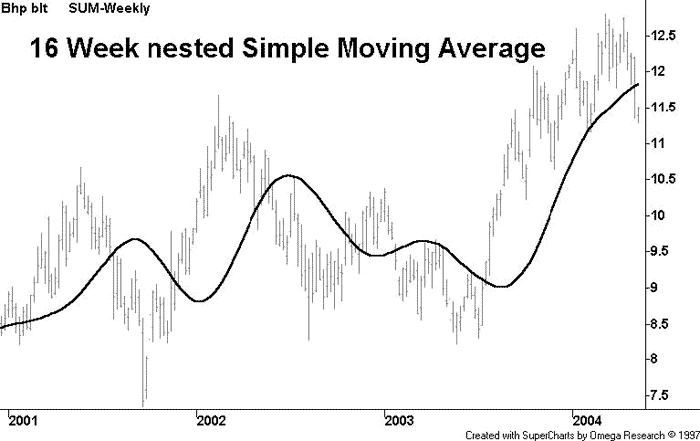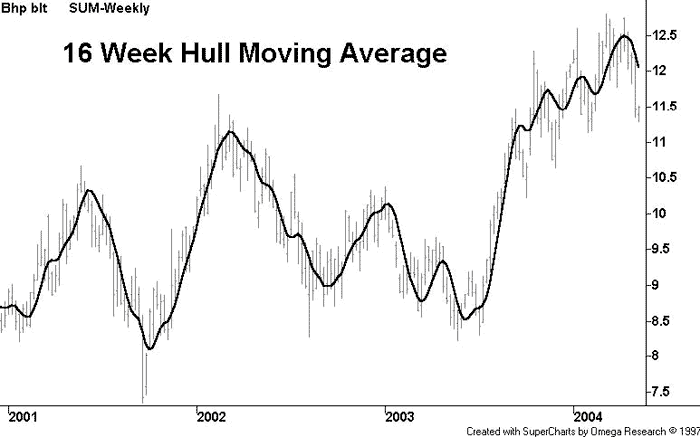# Hull Moving Average (HMA)

By Alan Hull

The Hull Moving Average solves the age old dilemma of making a moving average more responsive to current price activity whilst maintaining curve smoothness. In fact the HMA almost eliminates lag altogether and manages to improve smoothing at the same time. To understand how it achieves both of these opposing outcomes simultaneously we need to start with an easily understood frame of reference. The following chart contains a 16 week simple moving average which constantly lags the price activity and has poor smoothness.Firstly, solving the problem of curve smoothing can be done by taking an average of the average, i.e. 16 period SMA (16 period SMA (Price)). The bad news is that it causes a huge increase in lag as seen below.Solving the problem of lag is a bit more involved and requires an explanation with numbers rather than charts. Consider a series of 10 numbers from ‘0’ to ‘9’ inclusive and imagine that they are successive price points on a chart with 9 being the most recent price point at the right hand leading edge. If we take the 10 period simple average of these numbers then, not surprisingly, we will determine the midpoint of 4.5 which significantly lags behind the most recent price point of 9. Here’s the clever bit…first let’s halve the period of the average to 5 and apply it to the most recent numbers of 5,6,7,8, and 9, the result being the midpoint of 7.

 0 1 2 3 4 . 5 6 7 8 9 . . . . .. .. . . . . . . 4.5 . . 7 . .

Finally, to remove the lag we take the midpoint of 7 and add the difference between the two averages which equals 2.5 (7 – 4.5). This gives a final answer of 9.5 (7 + 2.5) which is a slight overcompensation. But this overcompensation is very handy because it offsets the lagging effect of the nested averaging. Hence the result of combining these 2 techniques is a near perfect balance between lag reduction and curve smoothing.The HMA manages to keep up with rapid changes in price activity whilst having superior smoothing over an SMA of the same period. The HMA employs weighted moving averages and dampens the smoothing effect (and resulting lag) by using the square root of the period instead of the actual period itself…as seen below.

Integer (Square Root (Period)) WMA [2 x Integer (Period/2) WMA (Price) – Period WMA (Price)]

The following formulas for the Hull Moving Average are for MetaStock and Supercharts but can be easily adapted for use with other charting programs that are capable of custom indicator construction.

MetaStock Formula

period:=Input(“period”,1,200,20);sqrtperiod:=Sqrt(period);Mov(2*Mov(C,period/2,W) – Mov(C,period,W),LastValue(sqrtperiod),W);

SuperCharts Formula

Input: period (Default value 20) waverage (2*waverage (close,period/2)-waverage (close,period), SquareRoot (Period))

A simple application for the HMA, given its superior smoothing, would be to employ the turning points as entry/exit signals. However it shouldn’t be used to generate crossover signals as this technique relies on lag.

Website: AlanHull.com